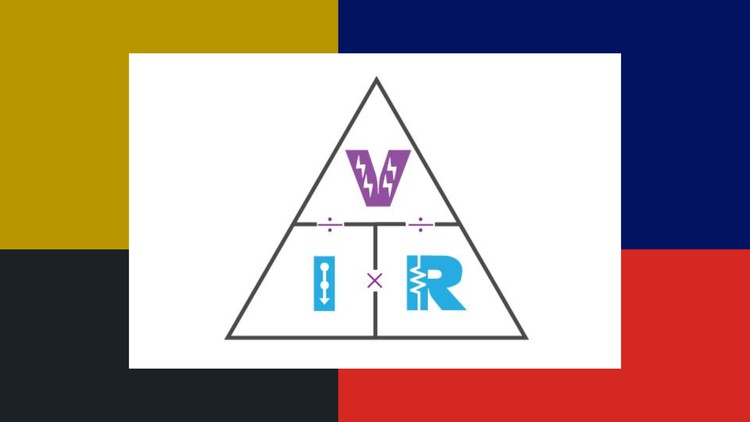# Fundamentals of Electrical and Electronics## Understand the Basic Concept of Electrical and Electronics Components

What you will learn

Current, Voltage, Energy and Power

Resistor, Inductor and Capacitor

Diode and Transistor

Ohm’s law and Kirchhoff Law

And …….. so much more!

Description

Dear Learners

Are you Preparing for Interview in Electronics and Electrical Industry? Don’t be stressed, take our  Electrical Engineering Course and prepare yourself for your Interview. In this Course You Understand the basic Concept of Electrical Engineering.

WHAT IS FOR YOU?

Knowledge of Electrical and Electronics is extremely valuable nowadays! In this Course you understand the Basic Concept of Electrical and Electronics Component.

• Here You Understand the Basic Concept of Current, Voltage, Energy and Power.
• Here You Understand the Resistor, Capacitor and Inductor.
• Here You Understand the Diode and Transistor
• Here you understand the The main laws governing currents and voltages in circuits that are Ohm’s Law and Kirchhoff Circuit Law.In Each Module you Understand the Theoretical and Practical Concept of the Component.

In the Present Days most of the material and courses available online tend to be in high-level and focused on applications , the main goal of this course is to explain the fundamental concepts of Electrical and Electronics, so that you understand that how circuits work. Every topic is addressed starting from basic working of the Component, and only later the acquired knowledge is used to explain how to design more complex circuits for different applications. In this way you understand all thing about that topic.

The Whole Course is Design in 4 module and there is a Quiz Section in Each Module.

I will be here for you every step of the way. If you have any questions about the course content,  you can always post a question in the course or send me a direct message.

Go ahead and click the enroll button.

Cheers,
Harish Kumar Maheshwari

## English

Language

Content

Introduction of the Course

Introduction of the Course

Fundamental concepts of Electricity

What is the Current ?

What is the Voltage ?

What Is the Energy ?

What is the Power ?

QUIZ 1

Fundamental Concept of Electrical components

What is Resistor ?

What is Capacitors ?

What is Inductor ?

QUIZ 2

Fundamental Concept of Electronics components

What is Diodes ?

What is Transistor ?

QUIZ 3

The main laws governing currents and voltages in circuits

What is the Ohm’s law ?

What is the Kirchhoff’s laws ?

QUIZ 4

Conclusion of the Course

Conclusion